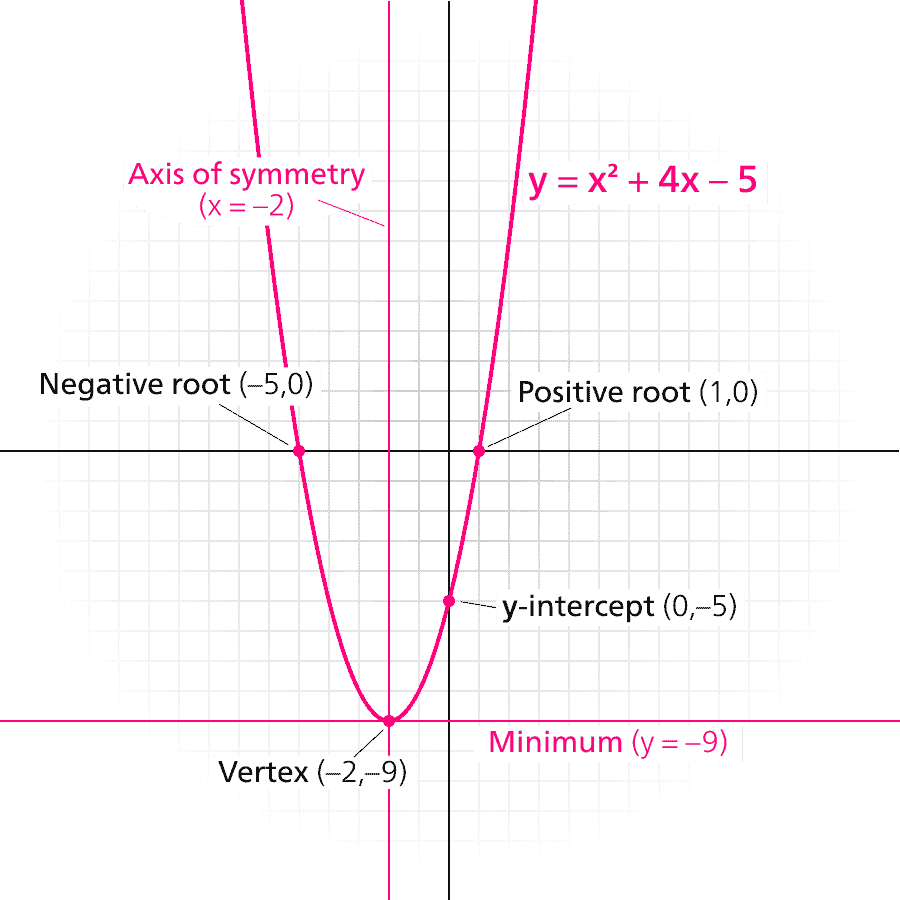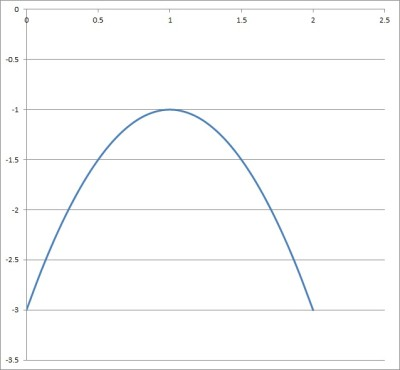# Domain & Range Of A Parabola (3 Key Ideas)

When working with a parabola, you may need to know the possible inputs (domain, or x-values) and outputs (range, or y-values).  This will involve finding the local minimum or maximum (vertex), along with figuring out concavity of the parabola.

So, what is the domain and range of a parabola?  The domain of a parabola y = ax2 + bx + c is the set of all real numbers – that is, any x-value is a valid input. The range of a convex parabola (for a > 0) is [vy, ∞), while the range of a concave parabola (for a < 0) is (-∞, vy], where vy = (4ac–b2)/4a is the y-coordinate of the parabola’s vertex.

The only things we really need to know are a (which tells us the concavity of a parabola) and vy (the y-coordinate of the vertex).  If we have the vertex form of a parabola, we can read both of these from the equation easily.

In this article, we’ll talk about the domain and range of a parabola, including how to find them from various forms of a parabola.

Let’s get started.

## Domain & Range Of A Parabola

Remember that the domain is the set of valid inputs (x-values), while the range is the set of possible outputs (y-values).A parabola has a vertex (local minimum for a > 0 or local maximum for a < 0), which helps us to find the range.

The domain is restricted when we have things like zero denominators or negative radicands.  The range can be restricted by domain restrictions, absolute value, etc.

## Domain Of A Parabola

The domain of a parabola is the entire set of real numbers, with no restrictions.  Since a parabola has no zero denominator or negative radicand, there is no restriction on the domain.

### Domain Of A Parabola In Standard Form

The domain of a parabola in standard form is the entire set of real numbers, with no restrictions.

Remember that a parabola in standard form has the equation

• y = ax2 + bx + c

where a, b, and c are real numbers and a is not zero.

There is no possibility of a zero denominator (there is no fraction) and no possibility of a negative radicand (there is no radical symbol).  So, there is no need to restrict the domain of the parabola.

### Domain Of A Parabola In Vertex Form

The domain of a parabola in vertex form is the entire set of real numbers, with no restrictions.

Remember that a parabola in vertex form has the equation

• y = a(x – h)2 + k

where a, h, and k are real numbers and a is not zero.  This form is helpful because the vertex of the parabola is given by the point (h, k), which we can easily find from the equation.

There is no possibility of a zero denominator (there is no fraction) and no possibility of a negative radicand (there is no radical symbol).  So, there is no need to restrict the domain of the parabola.

### Domain Of A Parabola In Factored Form

The domain of a parabola in factored form is the entire set of real numbers, with no restrictions.

Remember that a parabola in factored form has the equation

• y = a(x – r)(x – s)

where a is a nonzero real number and r, s are complex numbers.  The values r and s are values where the parabola is equal to zero (where the graph intersects the y-axis if r and s are real).

There is no possibility of a zero denominator (there is no fraction) and no possibility of a negative radicand (there is no radical symbol).  So, there is no need to restrict the domain of the parabola.

## Range Of A Parabola

The range of a parabola depends on two values:

• the value of a, the quadratic coefficient of the equation for the parabola
• the value of vy, the y-coordinate of the vertex of the parabola

There are two basic cases:

1. a > 0

If a > 0, then the parabola is convex (concave up), and the range is [vy, ∞).

• a < 0,

If a < 0, then the parabola is concave (concave down), and the range is (-∞, vy].

Note that we can find the value of vy for a parabola in standard form, vertex form, or factored form.  Let’s start with standard form.

### Range Of A Parabola In Standard Form

The range of a parabola in standard form is:

• [(4ac – b2)/4a, ∞) for a > 0
• (-∞, (4ac – b2)/4a] for a < 0

Remember that a parabola in standard form has the equation

• y = ax2 + bx + c

where a, b, and c are real numbers and a is not zero.

Let’s look at some examples.

#### Example 1: Range Of A Parabola In Standard Form

Consider the parabola y = 3x2 – 6x + 5, which is given in standard form.  Then we have a = 3, b = -6, and c = 5.

To find the y-coordinate of the vertex of this parabola, we use the formula:

• vy = (4ac – b2) / 4a
• vy = (4(3)(5) – (-6)2) / 4(3)
• vy = (60 – 36) / 12
• vy = (24) / 12
• vy = 2

So, the y-coordinate of the vertex is vy = 2.  Since a > 0 (a = 3), we know that the range of the parabola is [vy, ∞).

So, [2, ∞) is the range of the parabola – you can see its graph below.

#### Example 2: Range Of A Parabola In Standard Form

Consider the parabola y = -2x2 + 4x – 3, which is given in standard form.  Then we have a = -2, b = 4, and c = -3.

To find the y-coordinate of the vertex of this parabola, we use the formula:

• vy = (4ac – b2) / 4a
• vy = (4(-2)(-3) – 42) / 4(-2)
• vy = (24 – 16) / -8
• vy = (8) / -8
• vy = -1

So, the y-coordinate of the vertex is vy = -1.  Since a < 0 (a = -2), we know that the range of the parabola is (-∞, vy].

So, (-∞, -1] is the range of the parabola – you can see its graph below.The parabola y = y = -2x2 + 4x – 3 has a range of (-∞, -1].

### Range Of A Parabola In Vertex Form

The range of a parabola in vertex form is:

• [k, ∞) for a > 0
• (-∞, k] for a < 0

Remember that a parabola in vertex form has the equation

• y = a(x – h)2 + k

where a, h, and k are real numbers and a is not zero.  Also, (h, k) gives us the coordinates of the vertex, so k is the y coordinate of the vertex (that is, vy = k).

Let’s look at some examples.

#### Example 1: Range Of A Parabola In Vertex Form

Consider the parabola y = 2(x – 1)2 + 4, which is given in vertex form.  Then we have a = 2, h = 1, and k = 4.

To find the y-coordinate of the vertex of this parabola, we use the formula:

• vy = k
• vy = 4

So, the y-coordinate of the vertex is vy = 4.  Since a > 0 (a = 2), we know that the range of the parabola is [vy, ∞).

So, [4, ∞) is the range of the parabola – you can see its graph below.

#### Example 2: Range Of A Parabola In Vertex Form

Consider the parabola y = -4(x + 3)2 – 5, which is given in vertex form.  Then we have a = -4, h = -3, and k = -5.

To find the y-coordinate of the vertex of this parabola, we use the formula:

• vy = k
• vy = -5

So, the y-coordinate of the vertex is vy = -5.  Since a < 0 (a = -4), we know that the range of the parabola is (-∞, vy].

So, (-∞, -5] is the range of the parabola – you can see its graph below.

### Range Of A Parabola In Factored Form

The range of a parabola in factored form is:

• [-a(r – s)2/4, ∞) for a > 0
• (-∞, -a(r – s)2/4] for a < 0

Remember that a parabola in factored form has the equation

• y = a(x – r)(x – s)

where a, r, and s are real numbers and a is not zero.  Also, r and s give us the zeros of the quadratic (if r and s are real, they tell us the x-coordinates where the parabola intersects the x-axis).

Let’s look at some examples.

#### Example 1: Range Of A Parabola In Factored Form

Consider the parabola y = 6(x – 1)(x – 5), which is given in vertex form.  Then we have a = 6, r = 1, and s = 5.

To find the y-coordinate of the vertex of this parabola, we use the formula:

• vy = -a(r – s)2/4
• vy = -6(1 – 5)2/4
• vy = -6(-4)2/4
• vy = -6(16)/4
• vy = -96/4
• vy = -24

So, the y-coordinate of the vertex is vy = -24.  Since a > 0 (a = 6), we know that the range of the parabola is [vy, ∞).

So, [-24, ∞) is the range of the parabola – you can see its graph below.

#### Example 2: Range Of A Parabola In Factored Form

Consider the parabola y = -3(x – 2)(x – 10), which is given in vertex form.  Then we have a = -3, r = 2, and s = 10.

To find the y-coordinate of the vertex of this parabola, we use the formula:

• vy = -a(r – s)2/4
• vy = -(-3)(2 – 10)2/4
• vy = 3(-8)2/4
• vy = 3(64)/4
• vy = 192/4
• vy = 48

So, the y-coordinate of the vertex is vy = 48.  Since a < 0 (a = -3), we know that the range of the parabola is [vy, ∞).

So, (-∞, 48] is the range of the parabola – you can see its graph below.

## Conclusion

Now you know how to find the domain and range of a parabola, depending on which form of a parabola you are given (standard, vertex, or factored).

You can learn about the axis of symmetry for a parabola here.

You can learn about the focus of a parabola (and what it tells you) here.

You can learn how to find the domain and range of a polynomial here.

You can learn about the related concept of hyperbola (and how to graph them) here.